### Home > CALC > Chapter Ch7 > Lesson 7.1.3 > Problem7-31

7-31.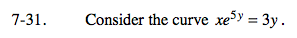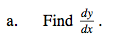Implicitly differentiate.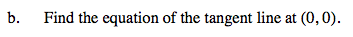Notice that the derivative you found in part (a) has both x- and y-values.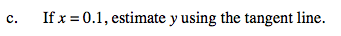Since x = 0.1 is easier to evaluate in a linear equation than in the curve shown above, use the tangent line to estimate the y-value at x = 0.1.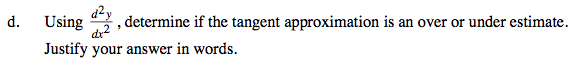$\text{When finding }\frac{d^{2}y}{dx^{2}}, \text{ remember to substitute your answer in part (a)}$

The sign of the 2nd derivative at (0,0) will determineif the tangent line is above or below the curve.

When a function is concave up, the tangent line will be below the curve.
When it is concave down, the tangent line will be above the curve.
Verify these statements by sketching a few examples.